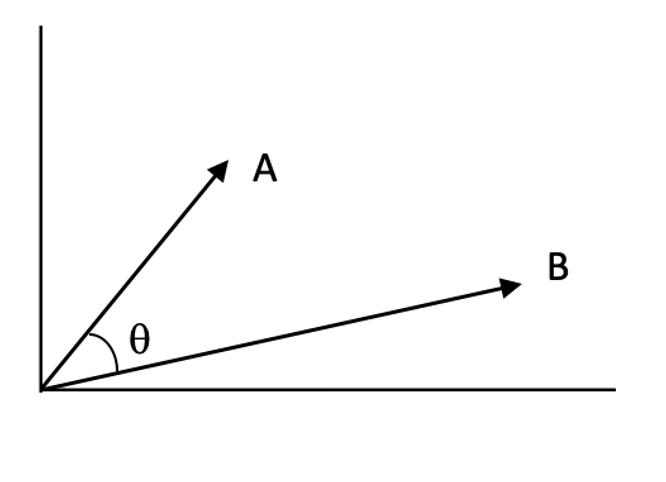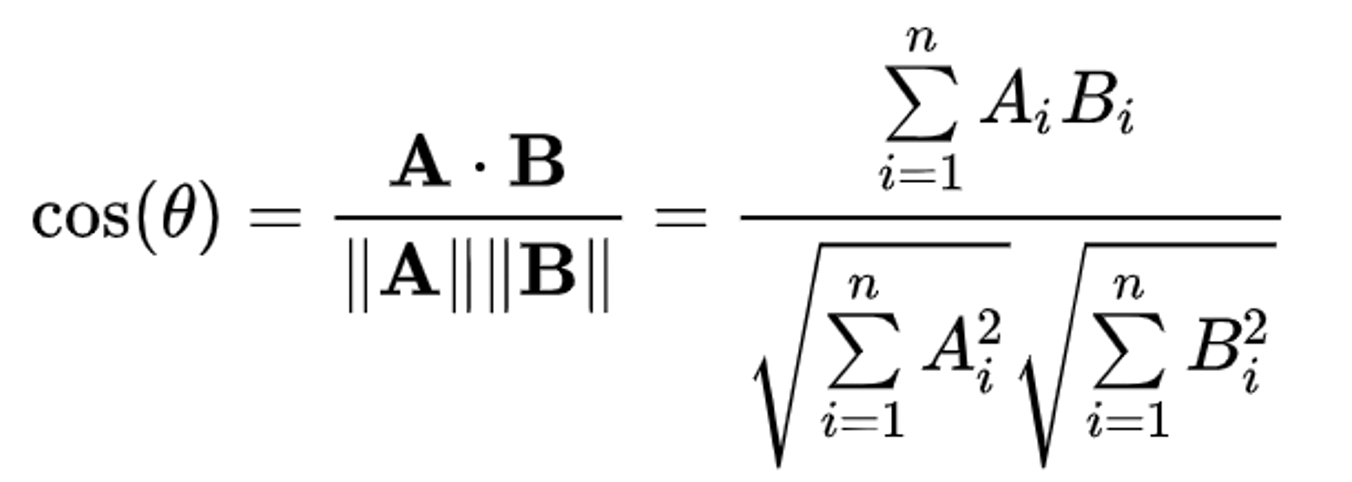• 余弦相似度python
2020-05-06 16:46:22
import numpy as np
import re

def get_word_vector(s1, s2):
"""
:param s1: 句子1
:param s2: 句子2
:return: 返回中英文句子切分后的向量
"""

# 把句子按字分开，中文按字分，英文按单词，数字按空格
regEx = re.compile('[\\W]*')
res = re.compile(r"([\u4e00-\u9fa5])")

p1 = regEx.split(s1.lower())
str1_list = []
for str in p1:
if res.split(str) == None:
str1_list.append(str)
else:
ret = res.split(str)
for ch in ret:
str1_list.append(ch)
# print(str1_list)

p2 = regEx.split(s2.lower())
str2_list = []
for str in p2:
if res.split(str) == None:
str2_list.append(str)
else:
ret = res.split(str)
for ch in ret:
str2_list.append(ch)
# print(str2_list)

list_word1 = [w for w in str1_list if len(w.strip()) > 0]  # 去掉为空的字符
list_word2 = [w for w in str2_list if len(w.strip()) > 0]  # 去掉为空的字符

# 列出所有的词,取并集
key_word = list(set(list_word1 + list_word2))
# 给定形状和类型的用0填充的矩阵存储向量
word_vector1 = np.zeros(len(key_word))
word_vector2 = np.zeros(len(key_word))

# 计算词频
# 依次确定向量的每个位置的值
for i in range(len(key_word)):
# 遍历key_word中每个词在句子中的出现次数
for j in range(len(list_word1)):
if key_word[i] == list_word1[j]:
word_vector1[i] += 1
for k in range(len(list_word2)):
if key_word[i] == list_word2[k]:
word_vector2[i] += 1

# 输出向量
return word_vector1, word_vector2

def cos_dist(vec1, vec2):
"""
:param vec1: 向量1
:param vec2: 向量2
:return: 返回两个向量的余弦相似度
"""
dist1 = float(np.dot(vec1, vec2) / (np.linalg.norm(vec1) * np.linalg.norm(vec2)))
return dist1

v1,v2 = get_word_vector('字符串1','字符串2')
a=cos_dist(v1,v2)
print(a)


原文：https://blog.csdn.net/weixin_44208569/article/details/90315904

更多相关内容
• 余弦相似度，又称为余弦相似性，是通过计算两个向量的夹角余弦值， 来评估他们的相似度。 余弦相似度将向量根据坐标值，绘制到向量空间中，如常见的二维空间。 余弦相似度衡量的是2个向量间的夹角大小，通过夹角的...
• 余弦相似度 pythonWhat is cosine similarity?什么是余弦相似度？ Cosine similarity measures the similarity between two vectors by calculating the cosine of the angle between the two vectors. 余弦相似度...

余弦相似度 python

What is cosine similarity?

什么是余弦相似度？

Cosine similarity measures the similarity between two vectors by calculating the cosine of the angle between the two vectors.

余弦相似度通过计算两个向量之间的角度的余弦值来衡量两个向量之间的相似度。

Cosine similarity is one of the most widely used and powerful similarity measure in Data Science. It is used in multiple applications such as finding similar documents in NLP, information retrieval, finding similar sequence to a DNA in bioinformatics, detecting plagiarism and may more.

余弦相似度是数据科学中使用最广泛，功能最强大的相似度之一。 它可用于多种应用程序，例如在NLP中查找相似的文档，信息检索，在生物信息学中查找与DNA相似的序列，检测抄袭等等。

Cosine similarity is calculated as follows,

余弦相似度计算如下：Angle between two 2-D vectors A and B (Image by author)calculation of cosine of the angle between A and B

Why cosine of the angle between A and B gives us the similarity?

为什么A和B之间的夹角余弦会给我们相似性？

If you look at the cosine function, it is 1 at theta = 0 and -1 at theta = 180, that means for two overlapping vectors cosine will be the highest and lowest for two exactly opposite vectors. You can consider 1-cosine as distance.

如果查看余弦函数，则在theta = 0处为1，在theta = 180处为-1，这意味着对于两个重叠的向量，余弦将是两个完全相反的向量的最高和最低值。 您可以将1-cosine作为距离。

How to calculate it in Python?

如何在Python中计算？

The numerator of the formula is the dot product of the two vectors and denominator is the product of L2 norm of both the vectors. Dot product of two vectors is the sum of element wise multiplication of the vectors and L2 norm is the square root of sum of squares of elements of a vector.

公式的分子是两个向量的点积，分母是两个向量的L2范数的乘积。 两个向量的点积是向量在元素上的乘积之和，而L2范数是向量的元素平方和的平方根。

We can either use inbuilt functions in Numpy library to calculate dot product and L2 norm of the vectors and put it in the formula or directly use the cosine_similarity from sklearn.metrics.pairwise. Consider two vectors A and B in 2-D, following code calculates the cosine similarity,

我们可以使用Numpy库中的内置函数来计算向量的点积和L2范数并将其放入公式中，也可以直接使用sklearn.metrics.pairwise中的cosine_similarity。 考虑二维中的两个向量A和B，下面的代码计算余弦相似度，

import numpy as npimport matplotlib.pyplot as plt# consider two vectors A and B in 2-DA=np.array([7,3])B=np.array([3,7])ax = plt.axes()ax.arrow(0.0, 0.0, A, A, head_width=0.4, head_length=0.5)plt.annotate(f"A({A},{A})", xy=(A, A),xytext=(A+0.5, A))ax.arrow(0.0, 0.0, B, B, head_width=0.4, head_length=0.5)plt.annotate(f"B({B},{B})", xy=(B, B),xytext=(B+0.5, B))plt.xlim(0,10)plt.ylim(0,10)plt.show()plt.close()# cosine similarity between A and Bcos_sim=np.dot(A,B)/(np.linalg.norm(A)*np.linalg.norm(B))print (f"Cosine Similarity between A and B:{cos_sim}")print (f"Cosine Distance between A and B:{1-cos_sim}")
# using sklearn to calculate cosine similarityfrom sklearn.metrics.pairwise import cosine_similarity,cosine_distancescos_sim=cosine_similarity(A.reshape(1,-1),B.reshape(1,-1))print (f"Cosine Similarity between A and B:{cos_sim}")print (f"Cosine Distance between A and B:{1-cos_sim}")
# using scipy, it calculates 1-cosinefrom scipy.spatial import distancedistance.cosine(A.reshape(1,-1),B.reshape(1,-1))

Proof of the formula

公式证明

Cosine similarity formula can be proved by using Law of cosines,

余弦相似度公式可以用余弦定律证明，

Consider two vectors A and B in 2-dimensions, such as,

考虑二维的两个向量A和B，例如，

Using Law of cosines,

利用余弦定律，

You can prove the same for 3-dimensions or any dimensions in general. It follows exactly same steps as above.

通常，您可以证明3维或任何尺寸的相同。 它遵循与上述完全相同的步骤。

Summary

概要

We saw how cosine similarity works, how to use it and why does it work. I hope this article helped in understanding the whole concept behind this powerful metric.

我们了解了余弦相似度如何工作，如何使用它以及为什么起作用。 我希望本文有助于理解这一强大指标背后的整个概念。

余弦相似度 python

展开全文• 也可以叫余弦相似度。 几何中夹角余弦可用来衡量两个向量方向的差异，机器学习中借用这一概念来衡量样本向量之间的差异。 (1)在二维空间中向量A(x1,y1)与向量B(x2,y2)的夹角余弦公式： (2) 两个n维样本点a(x11,x12,...
• 计算两句话的余弦相似度 源文: 使用余弦相似度算法计算文本相似度 - 一二三冲鸭的文章 - 知乎 https://zhuanlan.zhihu.com/p/43396514 """ import math from typing import List, Dict, AnyStr import jieba def...

参考知乎上一二三冲鸭同学的博客，根据自己理解对他的方法再实现一遍～

"""
计算两句话的余弦相似度

源文: 使用余弦相似度算法计算文本相似度 - 一二三冲鸭的文章 - 知乎
https://zhuanlan.zhihu.com/p/43396514
"""
import math
from typing import List, Dict, AnyStr

import jieba

def separate(text_data: AnyStr) -> List:
ret = jieba.cut(text_data, cut_all=False)
return [word for word in ret if word not in ['，', '。']]

def text_to_num(all_data: Dict, sep: List) -> List:
for index in range(0, len(sep)):
sep[index] = all_data[sep[index]]
return sep

def summary(sep1: List, sep2: List) -> Dict:
all_data = {}
data = list(set(sep1 + sep2))
for index in range(0, len(data)):
all_data[data[index]] = index
return all_data

def to_feq(all_data: Dict, sep: List) -> List:
temp = [0 for i in range(0, len(all_data))]
for num in sep:
temp[num] += 1
return temp

def compute_cosine_similarity(params1: List, params2: List) -> float:
# 计算余弦相似度
denominator_l = 0
denominator_r = 0
molecular = 0
for index in range(0, len(params1)):
molecular += (params1[index] * params2[index])
denominator_l += params1[index] ** 2
denominator_r += params2[index] ** 2
return molecular / (math.sqrt(denominator_l) * math.sqrt(denominator_r))

def run():
text1 = "飓风跳跃星球"
text2 = "飓风跳过星球"
seg_list1: List = separate(text1)
print(f'seg_list1分词: ' + '/'.join(seg_list1))
seg_list2: List = separate(text2)
print(f'seg_list2分词: ' + '/'.join(seg_list2))

all_data: Dict = summary(seg_list1, seg_list2)
print(f'all_list: {all_data}')
seg_list1: List = text_to_num(all_data, seg_list1)
print(f'seg_list1转为数字: {seg_list1}')
seg_list2: List = text_to_num(all_data, seg_list2)
print(f'seg_list2转为数字: {seg_list2}')

seg_list1: List = to_feq(all_data, seg_list1)
print(f'seg_list1词频统计: {seg_list1}')
seg_list2: List = to_feq(all_data, seg_list2)
print(f'seg_list2词频统计: {seg_list2}')
ret: float = compute_cosine_similarity(seg_list1, seg_list2)
print(f'余弦相似度为: {ret}')

if __name__ == '__main__':
run()



参考博客:
使用余弦相似度算法计算文本相似度 - 一二三冲鸭的文章 - 知乎
https://zhuanlan.zhihu.com/p/43396514

展开全文• 余弦相似度简介 余弦相似度，又称为余弦相似性，是通过计算两个向量的夹角余弦值来评估他们的相似度。对于两个向量，可以想象成空间中的两条线段，都是从原点（[0, 0, ...]）出发，指向不同的方向。两条线段之间...

# 一.余弦相似度简介

余弦相似度，又称为余弦相似性，是通过计算两个向量的夹角余弦值来评估他们的相似度。对于两个向量，可以想象成空间中的两条线段，都是从原点（[0, 0, ...]）出发，指向不同的方向。两条线段之间形成一个夹角：如果夹角为0度，则意味着方向相同、线段重合；如果夹角为90度，意味着形成直角，方向完全不相似；如果夹角为180度，意味着方向正好相反。因此，可以通过夹角的大小，来判断向量的相似程度。夹角越小，就代表越相似。

对n维向量A，B，假设A= [A1, A2, ..., An] ，B= [B1, B2, ..., Bn] ，则A与B的夹角θ的余弦等于：

余弦值的范围在[-1,1]之间，值越趋近于1，代表两个向量的方向越接近；越趋近于-1，他们的方向越相反；接近于0，表示两个向量近乎于正交。

一般情况下，相似度都是归一化到[0,1]区间内，因此余弦相似度表示为 cosine_similarity = 0.5cosθ + 0.5

# 二.余弦相似度与欧式距离的区别

欧氏距离衡量的是空间各点的绝对距离，跟各个点所在的位置坐标直接相关；而余弦距离衡量的是空间向量的夹角，更加体现在方向上的差异，而不是位置。

余弦距离使用两个向量夹角的余弦值作为衡量两个个体间差异的大小。相比欧氏距离，余弦距离更加注重两个向量在方向上的差异。

欧氏距离和余弦距离各自有不同的计算方式和衡量特征，因此它们适用于不同的数据分析模型：

1.欧氏距离能够体现个体数值特征的绝对差异，所以更多的用于需要从维度的数值大小中体现差异的分析，如使用用户行为指标分析用户价值的相似度或差异。

2.余弦距离更多的是从方向上区分差异，而对绝对的数值不敏感，更多的用于使用用户对内容评分来区分兴趣的相似度和差异，同时修正了用户间可能存在的度量标准不统一的问题（因为余弦距离对绝对数值不敏感）。

## 2.1调整余弦相似度:

正因为余弦相似度在数值上的不敏感，会导致这样一种情况存在：

用户对内容评分，按5分制，X和Y两个用户对两个内容的评分分别为（1,2）和（4,5），使用余弦相似度得到的结果是0.98，两者极为相似。但从评分上看X似乎不喜欢2这个 内容，而Y则比较喜欢，余弦相似度对数值的不敏感导致了结果的误差，需要修正这种不合理性就出现了调整余弦相似度，即所有维度上的数值都减去一个均值，比如X和Y的评分均值都是3，那么调整后为（-2，-1）和（1,2），再用余弦相似度计算，得到-0.8，相似度为负值并且差异不小，但显然更加符合现实。

# 三.余弦相似度的python实现

方法一：

def cosine_similarity(x, y, dim=256):
xx = 0.0
yy = 0.0
xy = 0.0
for i in range(dim):
xx += x[i] * x[i]
yy += y[i] * y[i]
xy += x[i] * y[i]
xx_sqrt = xx ** 0.5
yy_sqrt = yy ** 0.5
cos = xy/(xx_sqrt*yy_sqrt)*0.5+0.5
return cos

方法二：

import numpy as np

def cosine_similarity(x,y):
num = x.dot(y.T)
denom = np.linalg.norm(x) * np.linalg.norm(y)
return num / denom

方法三：

def cosine_similarity(x, y, norm=False):
assert len(x) == len(y), "len(x) != len(y)"
zero_list =  * len(x)
if x == zero_list or y == zero_list:
return float(1) if x == y else float(0)

res = np.array([[x[i] * y[i], x[i] * x[i], y[i] * y[i]] for i in range(len(x))])
cos = sum(res[:, 0]) / (np.sqrt(sum(res[:, 1])) * np.sqrt(sum(res[:, 2])))

return 0.5 * cos + 0.5 if norm else cos 
展开全文•numpy 开发语言
• 假设有一组数据集，该数据集包含有多个数据特征，现在需要对其特征维度进行算法分析，判断相互之间的相似度。如果说，有了特征维度的数据，再结合余弦定理的算法进行向量化，分析起来倒比较方便。所以说，重点是找出...机器学习 推荐算法
• 文本聚类 文本聚类的一种实现，使用 k-means 进行聚类，并使用作为距离度量。 等等，什么？ 基本上，如果您有一堆文本文档，并且您想按相似性将它们分成 n 个组，那么您很幸运。 例子 为了测试这一点，我们可以查看...
• 两向量之间的余弦相似度Prerequisite: 先决条件： Defining a Vector using list 使用列表定义向量 Defining Vector using Numpy 使用Numpy定义向量 Cosine similarity is a metric used to measure how similar ...
• faiss常用的两个相似度搜索是L2欧氏距离搜索和余弦距离搜索（注意不是余弦相似度） 简单的使用流程： import faiss index = faiss.IndexFlatL2(d) # 建立L2索引,d是向量维度 index = faiss.IndexFlatIP(d) # 建立In机器学习 开发语言
• 余弦相似度用向量空间中两个向量夹角的余弦值作为衡量两个个体间差异的大小。余弦值越接近1，就表明夹角越接近0度，也就是两个向量越相似，这就叫”余弦相似性”。具体算法请看：...
• from math import sqrt import numpy as np def similarity(v1, v2): a=sqrt( np.dot(v1, v1)) b=sqrt ( np.dot(v2, v2)) if a==0 or b==0: return -1 cos_dis=np.dot (v1, v2) / (b * a) ...
• ## Python计算余弦相似度

万次阅读 多人点赞 2020-08-09 10:51:39
余弦相似度常用在文本分类、图片分类等应用中，来计算两个文本或两个图像之间的相似度。 本文主要介绍通过Python计算两个向量的余弦相似度
• 基于TF-IDF算法、余弦相似度算法实现相似文本推荐——文本相似度算法，主要应用于文本聚类、相似文本推荐等场景。设计说明使用jieba切词，设置自定义字典使用TF-IDF算法，找出文章的关键词；每篇文章各取出若干个...
• 余弦相似度 from sklearn.metrics.pairwise import cosine_similarity a = [[1, 3, 2,5,6,7], [2, 2, 1,3,4,3],[2,2,2,2,2,2]] b = cosine_similarity(a) print(b) from sklearn.metrics.pairwise import cosine_...
• ## python-余弦相似度

千次阅读 2020-05-11 16:08:09
余弦相似度相关系数 import jieba import math import numpy as np #——————————————————————————创建样本与分词————————————————————————————————...
• ## python余弦相似度

千次阅读 2019-03-26 14:40:04
余弦相似度 在NLP的任务里，会对生成两个词向量进行相似度的计算，常常采用余弦相似度公式计算。 余弦相似度用向量空间中两个向量夹角的余弦值作为衡量两个个体间差异的大小。余弦值越接近1，就表明夹角越接近0度，...
• Python计算余弦相似度的方法及时间比较 形如有一个矩阵ashape形如（4,99999）和bshape形如(32,99999)，a的每一行去和b的每一行做余弦相似度计算，output一个shape形如(4，32)的余弦相似度矩阵。 a = np.random....
• 通过共现矩阵和余弦相似度实现机器对单词的认知、python实现本文介绍的定义：一、语料库预处理二、单词的分布式表示三、单词的相似度四、相似单词排序 本文介绍的定义： 语料库、计数方法的目的、语料库预处理、单词...机器学习 nlp
• ## TF-IDF与余弦相似度

千次阅读 2020-12-17 17:34:20
在文本挖掘的预处理中，向量化之后一般都伴随着TF-IDF的处理，那么什么是TF-IDF，为什么一般我们要加这一步预处理呢？... “余弦相似度”是一种非常有用的算法，只要是计算两个向量的相似程度，都可以采用它。
• 利用余弦相似度做文本分类：在数学中余弦相似度的公式：cos(a,b)=a*b/(|a|+|b|),而在文本上，我们的余弦相似度通常是这样计算而成：(文本a,b共同出现的词条数目)/(文本a出现的词条数目+文本b出现的词条数目)处理两个...
• /usr/bin/python3 import kashgari from kashgari.embeddings import BERTEmbedding import logging import numpy as np from sklearn.metrics.pairwise import cosine_similarity import jieba import sys chinese...
• 1.余弦相似度可用来计算两个向量的相似程度 对于如何计算两个向量的相似程度问题，可以把这它们想象成空间中的两条线段，都是从原点（[0, 0, ...]）出发，指向不同的方向。两条线段之间形成一个夹角，如果夹角为0度...
• 文章目录自然语言处理系列三十一文本相似度算法余弦相似度Python代码实现总结 自然语言处理系列三十一 文本相似度算法 在自然语言处理中，我们经常需要判定两个东西是否相似。比如，在微博的热点话题推荐那里，我们...算法 人工智能 字符串 java...